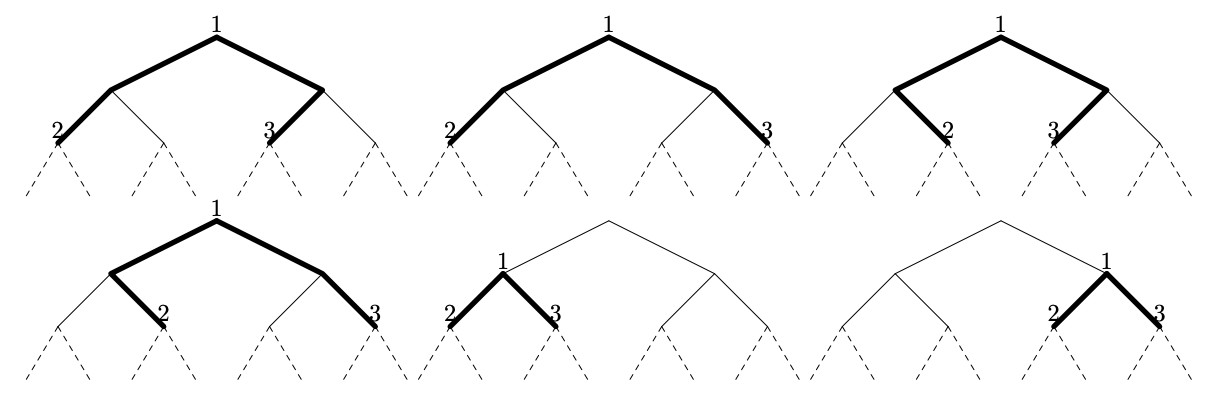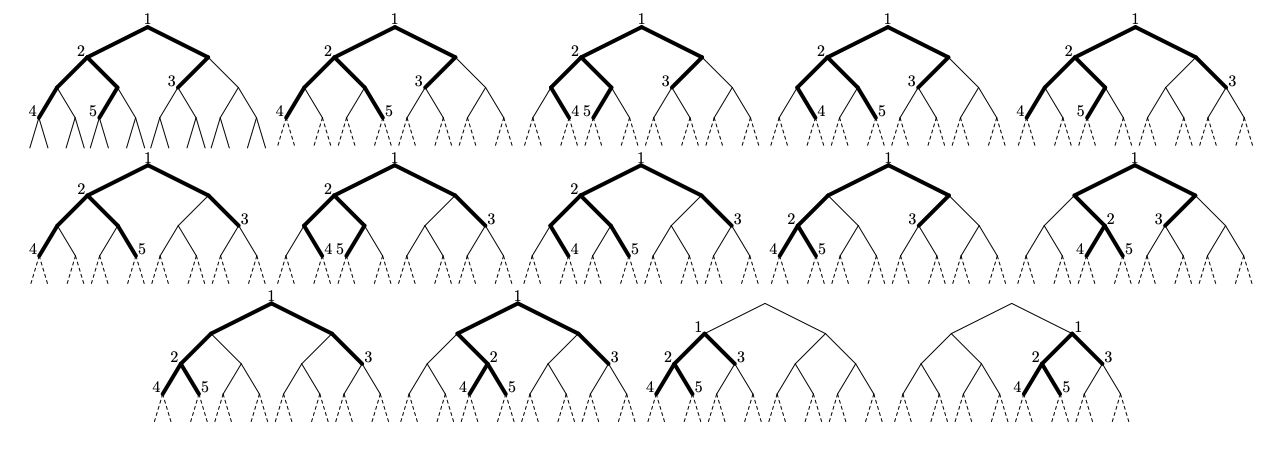시간 제한 메모리 제한 제출 정답 맞은 사람 정답 비율
2 초 256 MB 1 1 1 100.000%

## 문제

Vasya has got himself an infinite binary tree $T$, which can be formally described as follows: $T$ has infinitely many vertices, which are numbered starting from $1$. Each vertex of $T$ has two children --- left son and right son. The left son of the vertex $i$ is the vertex $2 i$, and the right son of the vertex $i$ is the vertex $2 i + 1$.

Also, Vasya has another binary tree $G$ (quite fortunately, a finite one). Every vertex of $G$ is either an internal vertex or a leaf. An internal vertex has a left son and a right son (and is called the parent of its children), and a leaf vertex has no children. Each vertex has exactly one parent, except for one vertex which has no parent; this vertex is called the root of the tree $G$.

Vasya would like to embed the tree $G$ in the infinite tree $T$. Formally, an embedding is defined as a function $f: V(G) \rightarrow V(T)$ (here $V(X)$ is the set of vertices of the tree $X$) with the following property: if the vertex $v$ is a left (alternatively: right) son of the vertex $u$ in $G$, then the vertex $f(v)$ must lie in the subtree of the left (alternatively: right) son of the vertex $f(u)$ in $T$.

Informally, an embedding is a way to put the vertices of $G$ somewhere on $T$ so that if we draw paths between all the embedded vertices and their children, the drawing looks like $G$ with some of the edges possibly extended downwards (looking at the pictures for the sample cases might help to understand the notion better).

Additionally, for each leaf vertex $v$ Vasya has chosen a number $h_v$ --- the height of the vertex of $T$ that $v$ should be embedded to (the height of a vertex is the number of edges between the vertex and the root of the tree). That is, $\text{height}(f(v)) = h_v$ must hold for every leaf $v$ of the tree $G$.

Now, Vasya wants to know the number of different embeddings of $G$ in $T$ such that each leaf $v$ is embedded to a vertex with height $h_v$. As the number may be quite large, find it modulo $10^9 + 7$.

## 입력

The first line contains one integer $n$ --- the number of vertices of $G$ ($1 \leq n \leq 2000$).

The next $n$ lines describe the tree $G$ and the numbers $h_v$.

If the $i$-th vertex is an internal vertex, then the first number in the $i$-th line will be $0$, followed by the numbers of its left and right sons respectively.

If the $i$-th vertex is a leaf, then the first number in the $i$-th line will be $1$, followed by the number $h_i$ for this leaf. All $h_i$ satisfy $0 \leq h_i \leq 10^9$.

It is guaranteed that the root of $G$ is the vertex $1$.

## 출력

Print one integer --- the number of appropriate embeddings modulo $10^9 + 7$.

## 예제 입력 1

3
0 2 3
1 2
1 2


## 예제 출력 1

6


## 예제 입력 2

5
0 2 3
0 4 5
1 2
1 3
1 3


## 예제 출력 2

14


## 예제 입력 3

3
0 2 3
1 0
1 0


## 예제 출력 3

0


## 예제 입력 4

1
1 0


## 예제 출력 4

1


## 힌트

All six possible embeddings for the first sample (note that the root of $G$ does not have to coincide with the root of $T$):All fourteen possible embeddings for the second sample: